# A Collection of Algorithms

#### pjk

Staff member
Here you can find algorithms to solve many different situations on the cube. If you have an algorithm you want to add, simply click on the link, and click on "Edit" at the top of the page, find the according case, and add it. Be sure to follow the syntax of the page before adding an algorithm. For example, to add an algorithm, find the case/picture, and add this at the bottom of the list:
Code:
* [insert your algorithm here]

Last edited:

#### Erik

##### Member
Originally posted by PJK@Mar 22 2007, 10:28 PM
Hello,
I am starting this thread so you can find nearly all permutation algorithms in 1 place. Hopefully we can get around 5-10 different algs to choose from for each case. If you have/use an algorithm that isn't listed yet, please reply with which one you are adding and I will add them. If you have an algorithm that isn't held in the same position as the picture, please mention that so I can denote that here. Thanks.

<hr>
Z Permutation
[*]M2 U' M' U2 M2 U2 M' U M2

V Permuation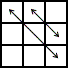• [R'UR'U'y'x R'U'R2x'U'R'UR'FRF]

<hr>
Y Permuation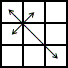• [R2 U' R' U R U' z' y' L' U' R U' R' U' L U y z]

<hr>
U Permuation : a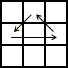• [y2 M2UMU2M'UM2]

<hr>
U Permuation : b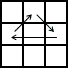• [y2 M2U'MU2M'U'M2]

<hr>
T Permuation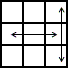• [R U R' U' R' F R2 U' R' U' R U R' F']

<hr>
R Permuation : a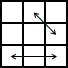• [y2z UR2U'R2UF'U'R'URUFU2]

<hr>
R Permuation : b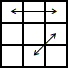• [R' U2 R U2 R' F R U R' U' R' F' R' U']

<hr>
N Permuation : a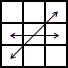• [zDR'UR2D'R(U'D)R'UR2D'RU'R]

<hr>
N Permuation : b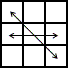• [zU'RD'R2'UR'(U'D)RD'R2UR'D'R']

<hr>
J Permuation : a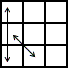• [y2 R' U2 R U R' Z' R2' U R' D R U']

<hr>
J Permuation : b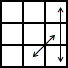• [R U2 R' U' R U2 L' U R' U' L]

<hr>
H Permuation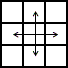• [M2 U' M2 U2' M2 U' M2]

<hr>
G Permuation : a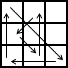• [R2' u R' U R' U' R u' R2 y' R' U R]

<hr>
G Permuation : b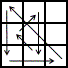• [y L' U' L y' R2' u R' U R U' R u' R2]

<hr>
G Permuation : c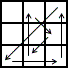• [y R2' u' R U' R U R' u R2 B U' B']

<hr>
G Permuation : d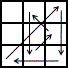• [y LU'RU2L'UR'y (R'L')U2(RL)]

<hr>
F Permuation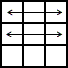• [R U' R' U R2 y R U R' U' x U' R' U R U2, or: yz R U R' U' R U2 z' y' R U R' U' y x L' U' L U L2 x']

<hr>
E Permuation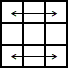• [y x R U' R' D R U R' D2 L' U L D L' U' L]

<hr>
A Permuation : a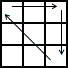• [x R' U R' D2 R U' R' D2 R2]

<hr>
A Permuation : b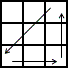• [y'x R2 D2 R U R' D2 R U' R]

<hr>
my algos

•zscuber73

#### pjk

Staff member
Updated.

I will go searching for algs soon#### Lt-UnReaL

##### Member
I didn't know you could do that with the U perms Erik! Also, we are missing the Z permutation. O_O

<hr>
• <hr>
Y Permuation• [F R U' R' U' R U R' F' R U R' U' R' F R F']
<hr>
R Permuation : a• [y' R2 B' R' U' R' U R B R' U2 R U2 R']
<hr>
R Permuation : b• [y R2 B2 U' R' U' R U R U B2 R U' R U]
<hr>
N Permuation : a• [y' L U' R U2 L' U R' L U' R U2 L' U R']
<hr>
N Permuation : b• [L' U R' U2' L U' L' R U R' U2' L U' R U']
<hr>
J Permuation : a• [y2 L' R' U2 R U R' U2 L U' R U ]
<hr>
J Permuation : b• [y2 R L U2 L' U' L U2 R' U L' U']
<hr>
x' R U' R' U D R' D U' R' U R D2' F x OR M2 U M2 U M' U2 M2 U2 M' U2

<hr>
F Permuation• [R' U R U' R2 F' U' F U x R U R' U' R2 B']
<hr>

•zscuber73

#### annon

##### Member
Could someone also list them in the basic UDFBRL notation?

#### pjk

Staff member
Updated, thanks L-t, sorry I missed it.

Annon, what do you mean? With no cube rotations? They are already in standard basic notation.

#### KJiptner

##### Member
JPermRUR' F' - RUR'U'R'-F-R2U'R'U'

(Similar to T-Perm)

•zscuber73

Staff member
Updated.

C

#### csfield

##### Guest
Here are mine:
<hr>
R Permutation : a• [R U2 R' U2 R B' R' U' R U R B R2' U]
<hr>
R Permutation : b• [R' U2 R U2 R' F R U R' U' R' F' R2 U']
<hr>
N Permutation : a• [R U' R' U l U F U' R' F' R U' R U l' U R']
<hr>
N Permutation : b• [R' U R U' R' F' U' F R U R' F R' F' R U' R]
<hr>
J Permutation : a• [y2 R' U2 R U R' U2' L U' R U L']
<hr>
<hr>
H Permutation• [R2' r2 U' L2 l2' U2 R2' r2 U' L2 l2']
<hr>
E Permutation• [y x R U' R' D R U R' u2 R' U R D R' U' R]
<hr>
Z Permutation : b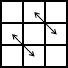• [U R' U' R U' R U R U' R' U R U R2 U' R' U]
<hr>
I got N b from Gungz' blog. N a is the F-B reflection with a double layer optimization. Bob Burton has that one on his site and attributes it to Stefan Pochmann. I like those N algs even though they are a little longer than the regular ones because they are finger-tricky and flow nicely. The H is the same alg as the M2 version just performed differently. I got that version from Gungz too and he has a nice video of it.

•zscuber73

#### KJiptner

##### Member
Thanks for sharing... interessting N's

#### dbeyer

##### Member
N Perm A
LU'L'ULFUF'L'U'LF'LFL'UL'
N Perm B
R'URU'R'F'U'FRUR'FR'F'RU'R

Edit
I just saw somebody added these, I don't use them, I do suggest them though!

#### Cubinator

##### Member
V Permutation• [R' U R' U' x2 y' R' U R' U' l R U' R' U R U]
<hr>
U Permutation : a• [B2 U' M' U2 M U' B2]
<hr>
U Permutation : b• [B2 U M' U2 M U B2]
<hr>
R Permutation : a• [R U2 R' U2 R B' R' U' R U l U R2 F]
<hr>
N Permutation : a• [R' U L' U2 R U' L R' U L' U2 R U' L]
<hr>
N Permutation : b• [R' U L' U2 R U' L R' U L' U2 R U' L]
<hr>
J Permutation : a• [y2 F2 L' U' r U2 l' U R' U' R U l U R2 F]
<hr>
H Permutation• [U' M2 U2 M2 U M2 U2 M2] - discovered that myself.
I have to go and will finsih the list later.

#### Lt-UnReaL

##### Member
Your U perms don't work. :/

#### Cubinator

##### Member
Whoops. I accidentally put B2 where it should have been U2. Fixed. Those aren't all the PLL algs, but the others that I have are already there.

#### pjk

Staff member
Updated. This is great. Please add yours if you haven't done so yet.

C

#### csfield

##### Guest
You posted one of my N's twice (once under J). Also the R b I posted is not a different algorithm but a correction of the typo (the last R' should be R2).

#### Cubinator

##### Member
Y Perm
F R U' R' F D R' (y x) R' U' R (z) R2 (y) L' d2

T Perm
F R U' R' U R U R2 F' R U R U' R'

R Perm b
R' U2 R U' (y') R' F R B' R' F' R (z x') R' U R'

N Perm a
R U' L d2 L' U L R' U' R U2 r' F l' U' (y2)
L U' R U2 L' U R' L U' R U2 L' U R' U'
The last alg for this case shouldn't be there. It's an N perm b alg.

J Perm a
R U' L' U R' U2 L U' L' U2' L

J Perm b
(y2) F2' R U R' (y) r2 (y') R U' R U R2'
L' U R U' L U2 R' U R U2' R'
The last alg for this case shouldn't be there either. It's a J perm a alg (and I don't think it's on the list)

H Perm
F2 M2 F2 U' F2 M2 F2 U- or (x) U2 M2 U2 B' U2 M2 U2 B
R2 U2 R2 U2 R2 U R2 U2 R2 U2 R2 U'
L R U2 L' R' F' B' U2 F B

Those are some algs you can add to your list. I'll find more later, time permitting. These were taken from cubestation (with the exception of the H perms, which are from Petrus' site, and one alg came from my head)

#### pjk

Staff member
Thanks, I updated. csfield, I think I corrected the problem, but not positive. Cubinator, I tried editing what you said too, let me know if I didn't do it correctly. When I get time I will go through and test all the algs. If you see a problem, please be specific on what it is. Thanks

#### Cubinator

##### Member
This alg [R' U L' U2 R U' L R' U L' U2 R U' L] is listed under N perm a and b, when it should only be a.

[y2 R' U2 R U R' U2' L U' R U L'] is listed as J perm b when it should be J perm a.